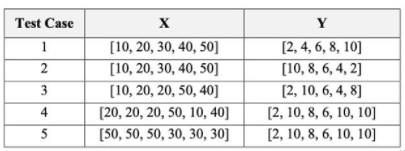# Python Programming

• The Spearman rank correlation is used to evaluate the relationship between two variables X and Y are monotonic. The rank correlation measures how closely related the ordering of one variable to the other variable is, with no regard to the actual values of the variables.

To calculate the Spearman rank correlation for Xand Y, ps(X, Y):

the values of X and Y are ranked in an ascending order respectively. The smallest value will be given a rank of 1, the next smaller value will be given a rank of 2, etc. If there are multiple entries with the same value, then each entry is ranked equal to the average position.

the rank difference for the i-th pair of x, and y, is then calculated andwhere N is the total pairs of data points and di is the rank difference for the i-th pair of data.

1. Create a function calculate speannan_rank correlation(X Y) that returns you the value of the rank correlation, given two sets of data X and Y.
2. Use your function on the test cases and print your results to one (1) decimal place